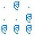## sábado, 27 de agosto de 2016

### Using the calculator in the terminal

Hi to all, this is a small issue I had the last days. Use a calculator in the terminal. I have been working and sometimes need to do an arithmetic operation so I decided to open  calc (Ubuntu Calculator Default). But I was wondering, there has to be a way to use a calculator in the CLI. So after some search in the engine I found this question in Ask Ubuntu and tried several options.

I will only write about the  best solution I found for my needs. In the question, you can find several solutions and probably you will find a different solution that will be best for you.

### CALC (Arbitrary precision calculator)

Calc is an arbitrary precision arithmetic system that uses a C-like language. Calc is useful as a calculator, an algorithm prototyper and as a mathematical research tool. More importantly, calc provides one with a machine independent means of computation. Calc comes with a rich set of builtin mathematical and programmatic functions.

If you want to install calc you can use the following command:

sudo apt-get install apcalc

#### 2 comentarios:

1.Este comentario ha sido eliminado por el autor.

2.Yes, I saw it as an option, but using echo and then pipe, didn't like it to much.